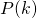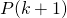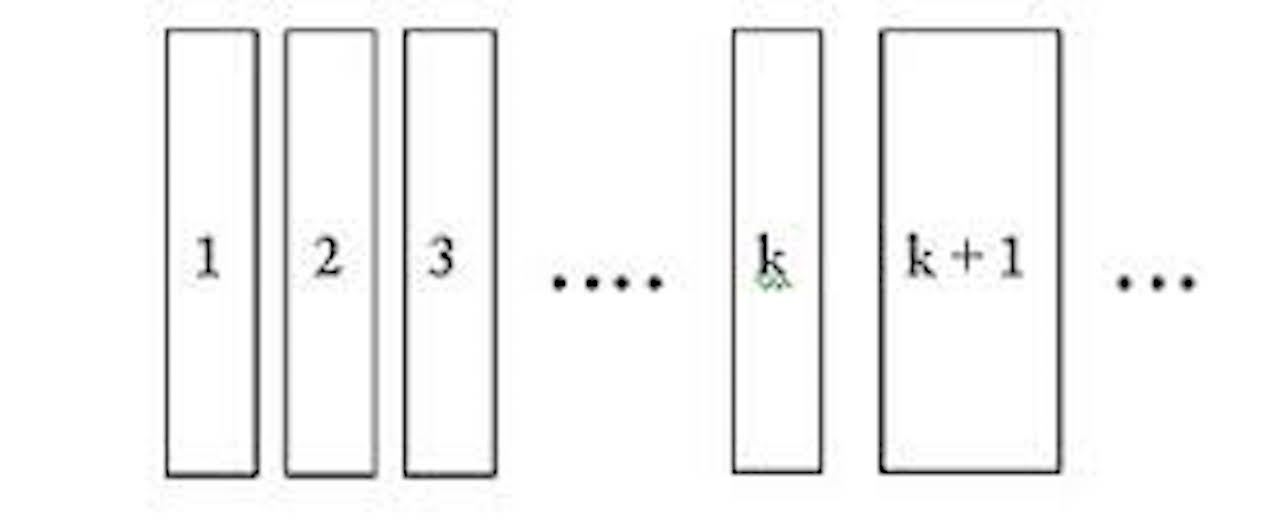# Intuition of Mathematical InductionMany students have asked me about Mathematical Induction, like whats the idea behind it. Especially since I always emphasize the importance of.

So lets try to make some sense of this long and mundane proof.

Our first step always seek to prove thatis true. And thenis our induction hypothesis, think of it as an assumption (so it might or might not be true). We then seek to proveis true with the use of. Should we succeed in proving it, we establish some relationship betweenand. But if we trace back,was a mere assumption. So where do we go from here?Credits: www.open.edu

If we trace back to, then we will create a domino effect like the above. Since we have thatis true definitely, using the proven relationship withand, we can establishis true. Here, we create a huge domino effect up to n.

Inquisitive students have asked me before, what if k is greater than n? Now that’s a much profound question to be answered. But curious students can google “Strong Mathematical Induction”.

Not readable? Change text.